A copper cylindrical tube has inner radius a and outer radius b. The resistivity is ρ. The resistance of the cylinder between the two ends is
• a)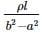• b)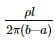• c)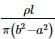• d)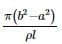### Related TestBs Academy Feb 13, 2022If one had considered a solid cylinder of radius b, one can suppose that it is made of two concentric cylinders of radius a and the outer part, joined along the length concentrically one inside the other.
If Ia and Ix are the currents flowing through the inner and outer cylinders
∵ Itotal = Ib = Ia + Ix
⇒ VRb = V/Ra + V/Rx
where Rb is the total resistance and Rx is the resistance of the tubular part.
∴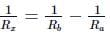But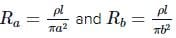∴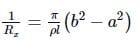∴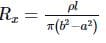If one had considered a solid cylinder of radius b, one can suppose that it is made of two concentric cylinders of radius a and the outer part, joined along the length concentrically one inside the other.If Ia and Ix are the currents flowing through the inner and outer cylinders Itotal = Ib = Ia + Ix⇒ VRb = V/Ra + V/Rxwhere Rb is the total resistance and Rx is the resistance of the tubular part.∴But∴∴
Do you know? How Toppers prepare for NEET Exam
With help of the best NEET teachers & toppers, We have prepared a guide for student who are preparing for NEET : 15 Steps to clear NEET Exam